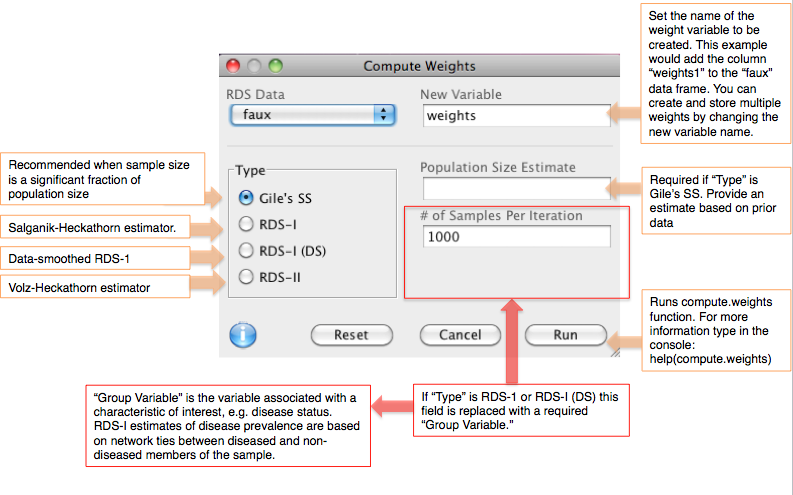Use the Compute Weights dialog under the Data menu to calculate sampling weights based on reported network size (degree). This dialog will store weights in your data set as a new variable (column) in the data set. Weights may be used for fitting a generalized linear model to the sample data. Several other dialogs in the software ask you to choose a weight type as part of their calculation, e.g. Frequency Estimates and Population Crosstabs. The methods available for calculating weights are described below. (In some dialogs, Arithmetic Mean is also listed as a weight type, which simply means that all members of the sample are weighted equally.)

• ''HCG' (Homophily Configuration Graph estimator): Recommended when the sample is a significant fraction of the target population and recruitment time is known. An estimate of the population size is required to use this estimator.
• Gile's SS (Sequential Sampler): Recommended when the sample is a significant fraction of the target population. It is based on the inclusion probabilities of members of the sample, which are based on reported network sizes (how many people a respondent knows within the target population). An estimate of the population size is required to use this estimator.
• RDS-I: The Salganik-Heckathorn estimator. RDS-I weights are calculated in reference to a particular categorical variable of interest (the Group Variable; it cannot be continuous). RDS-I calculations are based on the number of connections between "in-group" (e.g. infected) and "out-of-group" (e.g. uninfected) members of the sample (though more than two classes are possible). A Markov process is used to model population mixing, i.e. recruitment across groups, and generate an equilibrium estimate of group prevalence (e.g. infection rate).
• RDS-I (DS): Data-smoothed version of RDS-I. It is assumed that the Markov process is reversible.
• RDS-II: The Volz-Heckathorn estimator, a generalized Horvitz-Thomspon form. Like Gile's SS, it is based on inclusion probabilities for members of the sample, which are based on reported network sizes. It treats the sampling process as a random walk through the network of the target population.

For Gile's SS and RDS-II, weights will be unique for each reported network size. For RDS-I and RDS-I (DS), weights will be unique for each class of the Group Variable.